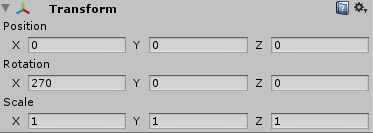# How to scale an object in editor with absolute or percentual values?

Maybe it is not possible but I’m looking since hours for an other way to change the size of an object.Is that the only possibility to change the size of an object?

Why can’t I say one object is 10cm width and the other one is 50% of that width?
I want to create objects in the editor not with scripts.
Or does Unity not support measurement/scale (cm, mm, m, feet …)?

Short answer is no, this is not built in.

To elaborate, Unity keeps the scale in arbitrary units as a way to keep things general. One rule of thumb is that one meter in Maya, imported at a scale of 1 is 1 Unity world unit. A Unity cube primitive is 1x1x1 unit and a plane primitive is 10x10 units. So by that logic, a Unity cube primitive at a scale of .01,.01,.01 is 1 cubic centimeter and a primitive plane at a scale of 100,1,100 (the y is irrelevant as it is a plane) is 1 square kilometer.

Hope that helps.

==

Assume “1” in unity means 1 meter, and have everything scale at 1, which is actually a percentage of the original size. I agree that it’s a cumbersome way but to get around that use blender to edit the model first, having blender set as 1=1 meter. If an object is not in meters then use import settings in Unity to convert the way you would otherwise such as 1 inch = 0.0254 meters.